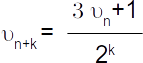# Collatz Sequence – part 1

May 29, 2018
By

Want to share your content on R-bloggers? click here if you have a blog, or here if you don't.

Collatz sequence is a well known suite mathematical suite, remaining unproved as far as I know.
Let’s play with it and discover some of its secrets.

To discover its behavior, we stop whenever we reach 1 as input, otherwise depending on parity of υn, we applyLet’s name 3υn + 1 the ODD rule, and the other the EVEN rule.

## Notation convention used in this document

All lowercase ASCII letters stands for natural integers.
The ‘o’ always means an odd integer.
The ‘e’ always means an even integer.

## Some trials …

Let’s start our analysis by playing with some elementary numbers, to get more familiar with the Collatz sequence generation suite.

``````#First number shown on each line is the starting number of the sequence
#Number in bracket is the number of steps to reach 1
> sapply(2:9, cn\$getCollatzSequence)
2 : 1 
3 : 10 5 16 8 4 2 1 
4 : 2 1 
5 : 16 8 4 2 1 
6 : 3 10 5 16 8 4 2 1 
7 : 22 11 34 17 52 26 13 40 20 10 5 16 8 4 2 1 
8 : 4 2 1 
9 : 28 14 7 22 11 34 17 52 26 13 40 20 10 5 16 8 4 2 1 
``````

Result 1.1: The conjecture is true for all 1 digit numbers.

Result 1.2: Each number 2k with k ≥ 1 will converge to the final value 1 in k steps.

Result 1.3: Each number 20q with q ∈ ℕ* will converge to the final value 1 in q + 6 steps.

LESSON LEARNT 1.1 The number of steps for an odd number to be reduced to1, is irregular. For example, 5 took 6 steps, whereas 3 took 8.
In the same time, a higher dimension of the input will bring a higher number of steps to converge to 1.

LESSON LEARNT 1.2: When proceeding to computation, we could end up earlier the processing, whenever we reached a number that is less than the starting input.
For example, consider starting number 9. Instead of doing the 19 computations required, we could have stopped at the first number in the suite
below the starting number 9, id est 7 that
appears in third place.

## Understanding the suite internal behavior and processing paths

### Basic understanding

The processing path is quite simple here. No shortcut, rigorous application of the υn + 1 suite rules.### A little bit more subtle understanding

Whenever we take an odd number multiply it by 3 and add 1, we end up with an even number. So in fact, each time we apply odd transformation, an even transformation will follow.This leads us to some insights. First, analyzing the possible paths on this graph, we have in fact only two possible loops. The first one will be named TIC and is given by (OE)+, the second one will be named TAC and is given by (OE{2,}). Syntax used here complies with PERL 5 regular expression syntax. This means that whenever we encounter an odd number we will apply either once and only once a parity condition, or several times.

Let’s see the consequences of this.

#### TAC case analysis

Here we alternate regularly ODD and EVEN suite rules for an unknown number of steps.INSIGHT 2.1: The TAC suite is naturally diverging to ∞ as we multiple each time by 3 / 2.

LESSON LEARNT 2.1: The TAC suite, whenever appearing in the computation, brings a natural integer as result, that will be greater than its input. So, it is an expansion factor.

#### TIC case analysis

Here we do not alternate regularly ODD and EVEN suite rules.
Instead we apply first and once and only once the ODD rule, and then apply at k times the EVEN rule, with k ≥ 2.INSIGHT 3.1: The TIC suite is converging to 0 with k → ∞. This means that the number we compute, will be lesser than the number we used as input.

LESSON LEARNT 3.1: The TIC suite, whenever appearing in the computation, brings a natural integer as result, that will be smaller than its input. So, it is a reduction factor.

#### Digit mutation analysis

It appears that Collatz sequence turns digits in a very strange way, due to its definition. All results can be summarize at a digit level with following figure.This graphs shows that the digit paths have well established cycles, and that we can consider 3 different clusters. First cluster is based on numbers ending with a digit 6. That’s also the cluster of numbers that are multiples of 10.
Second cluster is based on source numbers ending with a digit 4, and the third cluster based on numbers ending with a digit 8.

INSIGHT 4.1: Note that to enter cluster 2 you must have a digit division with tens being even and to enter cluster 1 or cluster 1 you must have a digit division with tens being odd.

INSIGHT 4.2: Note that each cluster have only one entry point and one exit point. Entry and exit point are unique for cluster 1 and cluster 3 and are distinct for cluster 2.

INSIGHT 4.3: Each digit belongs to one and only one cluster.

## Conclusion of part 1

So, if we want to prove the conjecture, then we just have to prove it for any number with digit 4 or 6 or 8 that are the entry points of each cluster. We’ll try this in second post to come.

R-bloggers.com offers daily e-mail updates about R news and tutorials about learning R and many other topics. Click here if you're looking to post or find an R/data-science job.
Want to share your content on R-bloggers? click here if you have a blog, or here if you don't.